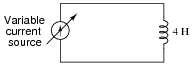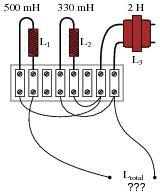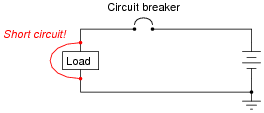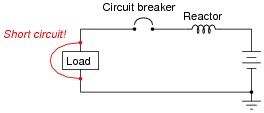# Inductors

## DC Electric Circuits

• #### Question 1

A 5 H inductor is subjected to an electric current that changes at a rate of 4.5 amps per second. How much voltage will be dropped by the inductor?

• #### Question 2

Suppose an inductor is connected to a variable current source, where the current is steadily increased at a rate of 1.5 amps per second. How much voltage will the 4 Henry inductor drop, and what will be the polarity of that drop? Remember, the direction of the arrow in a current source symbol points in the direction of conventional flow, not electron flow!In real life, an inductor will not drop the exact same amount of voltage that you will calculate here. Determine if the real voltage drop across such an inductor would be greater or less than predicted, and explain why.

• #### Question 3

Two 5 H inductors connected in series are subjected to an electric current that changes at a rate of 4.5 amps per second. How much voltage will be dropped across the series combination?

Now suppose that two 5 H inductors connected in parallel are subjected to the same total applied current (changing at a rate of 4.5 amps per second). How much voltage will be dropped by these inductors? Hint: the total current is divided evenly between the two inductors.

• #### Question 4

Suppose two 50 mH inductors are connected in parallel with each other. What will their combined inductance be, in Henrys? Explain your answer.

• #### Question 5

Calculate the total inductance in this collection of inductors, as measured between the two wires:Also, write a formula that calculates total inductance given the values of inductors L1, L2, and L3.

• #### Question 6

How large must inductor Lx be in order to provide a total inductance of 2.5 H in this network of inductors?• #### Question 7

Suppose a short-circuit were to develop in this electric power system:The purpose of the circuit breaker, of course, is to open the circuit automatically, to prevent damage to the power conductors. In a large electric power system, the magnitudes of such short-circuit currents can be enormous.

Large inductors, commonly called reactors, are often installed in series with power conductors in high-voltage power systems in order to “soften” the onset of short-circuit currents:Explain how the addition of a “reactor” helps to minimize the magnitude of the short-circuit current the breaker has to interrupt.

• #### Question 8

Small inductors often look like resistors, even to the extent that their values are designated by colored bands. Determine the values of the following inductors (express the tolerance as a percentage), based on their color codes:

Red, Grn, Brn, Gld
Wht, Org, Red, Sil
Grn, Gry, Blk
Vio, Blu, Org, Gld
• #### Question 9

When checked with an ohmmeter, how should a properly functioning inductor respond?

• #### Question 10

What happens to the inductance of an inductor as its core becomes saturated? Does the inductance value increase, decrease, or remain the same? Explain your answer.

• #### Question 11

Find one or two real inductors and bring them with you to class for discussion. Identify as much information as you can about your inductors prior to discussion:

Inductance (ideal)
Inductance (actual)
Winding resistance
Current rating
Type (iron core, air core, etc.)